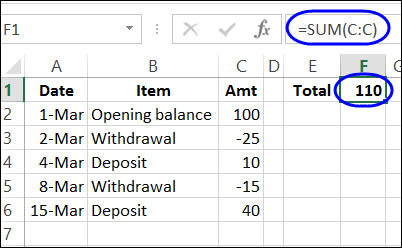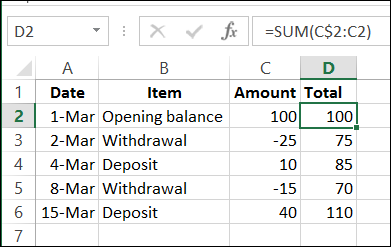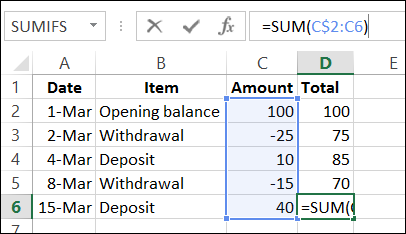## Sunday, January 4, 2015

### MS Excel, Create Running total with SUM

If you're using worksheet formulas, instead of a pivot table, there's nothing built in that will automatically create a running total for you. Fortunately, with a simple SUM formula, you can calculate the running total in each row, to see how your bank account is doing.

### Use the SUM Function

In this example, there are withdrawal and deposit amounts in cells C2:C6. We could use the SUM function to total the amounts in column C, and that would give us the current total.Instead, we'll use the SUM function in each row, to calculate the running total. We'll be able to see the total after each withdrawal and deposit.

The formula in cell D2 is: =SUM(C\$2:C2)That \$ sign locks the reference to row 2, at the start of the sum, so it doesn't change when the formula is copied down to cell D6.

The ending cell – C2 – is a relative reference, so the ending point changes for each row. For example, in cell D6, the formula shows C6 as the ending cell for the SUM formula.